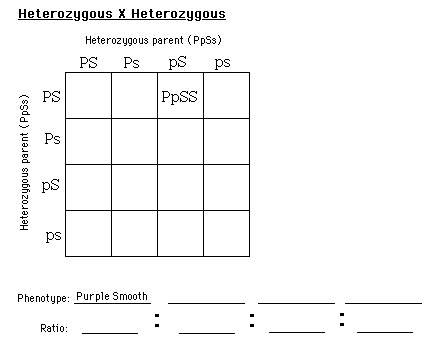Dihybrid Cross in Corn

 Dihybrid Cross in CornINTRODUCTION:

A dihybrid cross is a cross between individuals that involves two pairs of contrasting traits. Predicting the results of a dihybrid cross is more complicated than predicting the results of a monohybrid cross. All possible combinations of the four alleles from each parent must be considered.
We will examine a dihybrid cross involving both color and texture. Purple (P), is dominate to yellow (p), and smooth texture (S) is dominant to wrinkled (s). Both parent plants are heterozygous for both traits.
Review genetics and the use of Punnett squares in a biology text before doing this experiment.

MATERIALS:

Appropriate ear of corn.

You can purchase them from a biological supply, such as Carolina. You need a heterozygous X heterozygous 9:3:3:1, purple/yellow, starchy/sweet.

PROCEDURE:

Theoretical: First let us use a Punnett square to examine the theoretical outcome of the Heterozygous X Heterozygous dihybrid cross.

1. Fill in the Punnett square. Each box represents a genotype possibility for an offspring. Place the alleles donated by each parent in the corresponding box. One offspring has been done for you as an example. Now list the possible phenotypes in the spaces below the Punnett square. REMEMBER: a phenotype is how the offspring will look. If an individual’s genotype is heterozygous, the dominant trait will be expressed in the phenotype. There are four possible phenotypes for the offspring of this cross, and both traits are in each phenotype. One has been done for you as an example.

Now show the ratio of the phenotypes. For this, it is simply the number of possible individuals with each phenotype.Actual cross: Now we will make a count of an actual cross and compare the calculations of it’s phenotype ratios to the theoretical.

2. Obtain an ear of corn that is the result of a cross that was Heterozygous X Heterozygous for both traits. Copy the four phenotypes in the appropriate blanks, then count and record the number of kernels for each phenotype.

Now calculate the ratio for the cross. The phenotype with the least number of individuals you will call 1. Place the 1 in the space below the appropriate phenotype. Now divide the other count numbers by the number of individuals from the phenotype you called 1, and round your answers to the nearest whole number. Put the answers under the appropriate phenotypes.

 Phenotype: Number: Ratio: _____________ _____________ _____________ _____________ _______ _______ _______ _______ ______ : ______ : ______ : ______

Compare your results with the theoretical answers you obtained for the cross. Did you obtain a ratio in your experiment that was close to the theoretical?